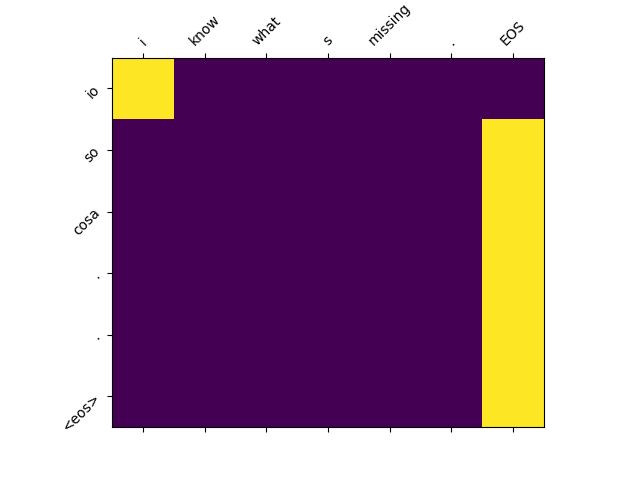# [seq2seq] Attention layer not able to align

I’m implementing a seq2seq word based model with Attention to translate from English to Italian, but my Attention mechanism does’t seems to work well. I’m using GRU-RNN and the Attention mechanism uses the dot product.
I get good training loss going down exponentially from 3.5 to 0.8 reaching the plateau, but my results are not so good. Following there’s a typical attention matrix I get (all my results are vary similar to this one). x-axes = english sentence, y-axes = italian prediction. It seems that the model is able to align correctly the first word of the sentence, but then it just focus on the end of sequence “EOS” and doesn’t align anymore. Any suggestion??I’ll show you my Decoder code:

``````class Decoder(nn.Module):
def __init__(self):
super(Decoder, self).__init__()

self.attention = AttentionDot()
self.embedding = nn.Embedding(PDec['output_size'], PEmb['embedding_size'], padding_idx=0)  # PDec['outputsize'] is because it is embedding the last word
self.gru = nn.GRU(PDec['input_size'], PDec['hidden_size'], num_layers=PDec['num_layers'], batch_first=True, dropout=PDec['dropout'])
self.out = nn.Linear(PDec['hidden_size'], PDec['output_size'])
self.log_softmax = nn.LogSoftmax(dim=2)

def forward(self, last_word_index, decoder_output, decoder_hidden, encoder_annotations, lengths):
# getting attention weights over encoder_annotations
focus_prob = self.attention(decoder_output, encoder_annotations, lengths) #[BxTx1]
context = torch.bmm(focus_prob.transpose(1,2), encoder_annotations.transpose(0,1)) #[Bx1xT] bmm [BxTx2*H] = [Bx1x2*H]

# concatenate context with last_char_index
last_word_index = last_word_index.type(dtypeL).squeeze(1)
last_word_index = self.embedding(last_word_index)
dec_input = torch.cat((context, last_word_index),2) #[Bx1x(2*H+Emb)]

decoder_output, decoder_hidden = self.gru(dec_input, decoder_hidden) # decoder_output = [Bx1xH], decoder_hidden = [num_layx1x(2*H+Emb)]
unnormalize_log_prob = self.out(decoder_output)
prob = self.log_softmax(unnormalize_log_prob) # [Bx1xalpLen]

return focus_prob, decoder_output, decoder_hidden, prob

def initFirstInputIndex(self, batch_size):
dec_in = L.SOS_token
dec_in = Variable(torch.ones(batch_size, 1, 1).fill_(dec_in).type(dtypeF)) #[Bx1x1]
return dec_in

def InitFirstHidden(self, batch_size):
hid = Variable(torch.zeros(PDec['num_layers'], batch_size, PEnc['hidden_size']).type(dtypeF))
return hid

def InitFirstOutput(self, batch_size):
out = Variable(torch.ones(batch_size, 1, PDec['hidden_size']).type(dtypeF))
return out
``````

and the Attention one:

``````class AttentionDot(nn.Module):
"""
attention with dot product
"""
def __init__(self):
super(AttentionDot, self).__init__()

self.linear_enc = nn.Linear(PEnc['hidden_size']*PAtt['encoder_directions'], PAtt['attention_size'], bias=False)
self.linear_dec = nn.Linear(PDec['hidden_size'], PAtt['attention_size'])

def forward(self, decoder_state, encoder_annotations, lengths):
att_decoder_state = self.linear_dec(decoder_state).transpose(1,2) #[BxAttx1]
att_encoder_annotations = self.linear_enc(encoder_annotations).transpose(0,1) #[BxTxAtt]

# attention scores
scores = torch.bmm(att_encoder_annotations, att_decoder_state) #[BxTx1]

# prob distribution over scores

return focus_prob  #[BxTx1]

"""
masked softmax for batches with shifted unnormalize probabilities for stability.
"""

# get ones where x values are non-zero

# find max values and shift to deal with numerical instability
maxes, _ = torch.max(x, dim=1, keepdim=True)
shiftx = x - maxes